# MobileNet-V2的来源¶

Mobilenet-V1的出现推动了移动端的神经网络发展。但MobileNet V1主要使用的Depthwise Conv（深度可分离卷积）虽然降低了9倍的计算量，但遗留了一个问题是我们在实际使用的时候训练完发现kernel有不少是空的。当时我们认为，Depthwise每个kernel dim相对于普通Conv要小得多，过小的kernel_dim, 加上ReLU的激活影响下，使得神经元输出很容易变为0，然后就学废了（因为对于ReLU来说，值为0的地方梯度也为0）。我们还发现，这个问题在定点化低精度训练的时候会进一步放大。所以为了解决这一大缺点，MobileNet-V2横空出世。

# MobileNet-V2的创新点¶

## 反残差模块¶

MobileNet V1没有很好的利用残差连接，而残差连接通常情况下总是好的，所以MobileNet V2加上残差连接。先看看原始的残差模块长什么样，如Figure3左图所示：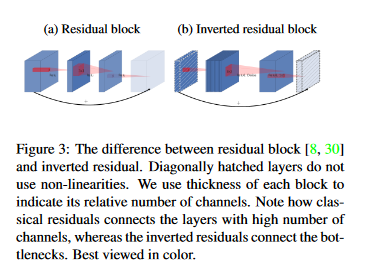# 网络结构¶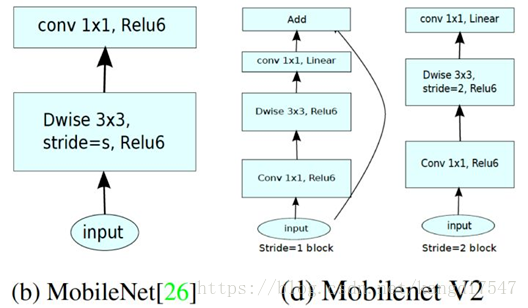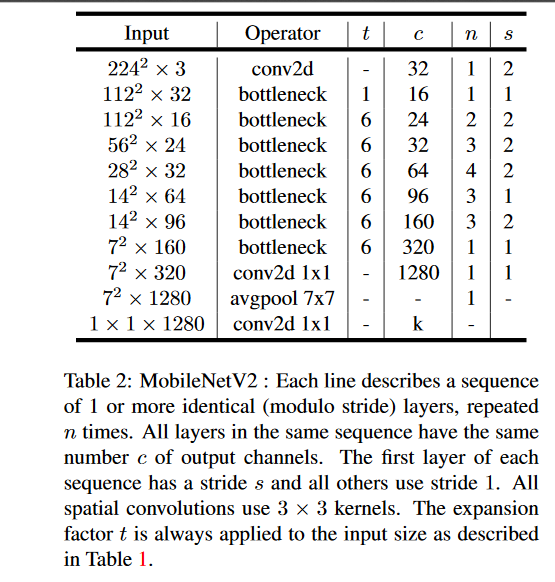# 实验结果¶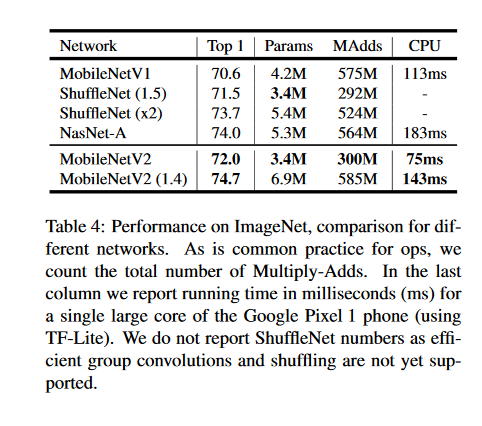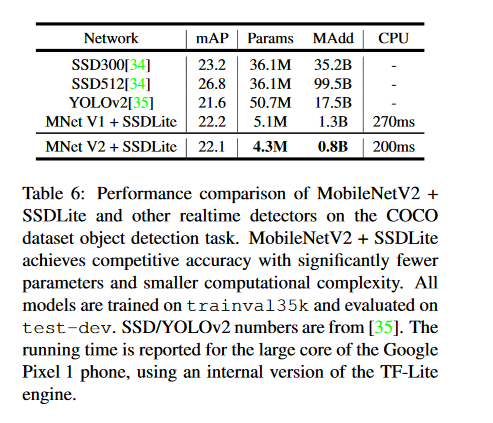# 总结¶

• 本文提出了一个新的反残差模块并构建了MobileNet V2，效果比MobileNet V1更好，且参数更少。
• 本文最难理解的其实是反残差模块中最后的线性映射，论文中用了很多公式来描述这个思想，但是实现上非常简单，就是在 MobileNet V1微结构(bottleneck)中第二个$1\times 1$卷积后去掉 ReLU6。对于低维空间而言，进行线性映射会保存特征，而非线性映射会破坏特征。

# Pytorch代码实现¶

class Block(nn.Module):
'''expand + depthwise + pointwise'''
def __init__(self, in_planes, out_planes, expansion, stride):
super(Block, self).__init__()
self.stride = stride

planes = expansion * in_planes
self.conv1 = nn.Conv2d(in_planes, planes, kernel_size=1, stride=1, padding=0, bias=False)
self.bn1 = nn.BatchNorm2d(planes)
self.conv2 = nn.Conv2d(planes, planes, kernel_size=3, stride=stride, padding=1, groups=planes, bias=False)
self.bn2 = nn.BatchNorm2d(planes)
self.conv3 = nn.Conv2d(planes, out_planes, kernel_size=1, stride=1, padding=0, bias=False)
self.bn3 = nn.BatchNorm2d(out_planes)

self.shortcut = nn.Sequential()
if stride == 1 and in_planes != out_planes:
self.shortcut = nn.Sequential(
nn.Conv2d(in_planes, out_planes, kernel_size=1, stride=1, padding=0, bias=False),
nn.BatchNorm2d(out_planes),
)

def forward(self, x):
out = F.relu(self.bn1(self.conv1(x)))
out = F.relu(self.bn2(self.conv2(out)))
out = self.bn3(self.conv3(out))
out = out + self.shortcut(x) if self.stride==1 else out
return out

class MobileNetV2(nn.Module):
# (expansion, out_planes, num_blocks, stride)
cfg = [(1,  16, 1, 1),
(6,  24, 2, 1),  # NOTE: change stride 2 -> 1 for CIFAR10
(6,  32, 3, 2),
(6,  64, 4, 2),
(6,  96, 3, 1),
(6, 160, 3, 2),
(6, 320, 1, 1)]

def __init__(self, num_classes=10):
super(MobileNetV2, self).__init__()
# NOTE: change conv1 stride 2 -> 1 for CIFAR10
self.conv1 = nn.Conv2d(3, 32, kernel_size=3, stride=1, padding=1, bias=False)
self.bn1 = nn.BatchNorm2d(32)
self.layers = self._make_layers(in_planes=32)
self.conv2 = nn.Conv2d(320, 1280, kernel_size=1, stride=1, padding=0, bias=False)
self.bn2 = nn.BatchNorm2d(1280)
self.linear = nn.Linear(1280, num_classes)

def _make_layers(self, in_planes):
layers = []
for expansion, out_planes, num_blocks, stride in self.cfg:
strides = [stride] + *(num_blocks-1)
for stride in strides:
layers.append(Block(in_planes, out_planes, expansion, stride))
in_planes = out_planes
return nn.Sequential(*layers)

def forward(self, x):
out = F.relu(self.bn1(self.conv1(x)))
out = self.layers(out)
out = F.relu(self.bn2(self.conv2(out)))
# NOTE: change pooling kernel_size 7 -> 4 for CIFAR10
out = F.avg_pool2d(out, 4)
out = out.view(out.size(0), -1)
out = self.linear(out)
return out


# 推荐阅读¶